### IMO Shortlist 1994 problem A3

Kvaliteta:
Avg: 0,0
Težina:
Avg: 7,0
Let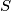$S$ be the set of all real numbers strictly greater than −1. Find all functions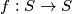$f: S \to S$ satisfying the two conditions:

(a)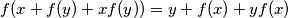$f(x + f(y) + xf(y)) = y + f(x) + yf(x)$ for all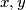$x, y$ in$S$;

(b)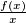$\frac {f(x)}{x}$ is strictly increasing on each of the two intervals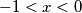$- 1 < x < 0$ and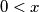$0 < x$.
Izvor: Međunarodna matematička olimpijada, shortlist 1994